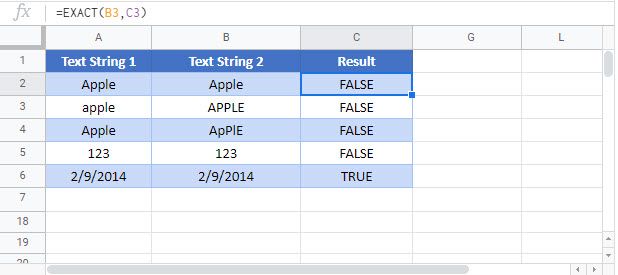# EXACT Function Examples – Excel & Google Sheets

This Tutorial demonstrates how to use the Excel EXACT Function in Excel to test if cells are exactly the same.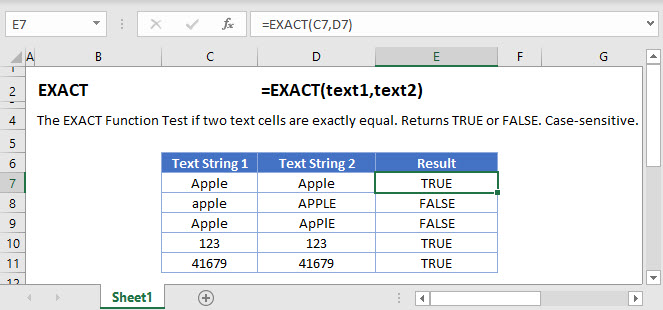## EXACT Function Overview

The EXACT Function Test if two text cells are exactly equal. Returns TRUE or FALSE. Case-sensitive.

To use the EXACT Excel Worksheet Function, select a cell and type: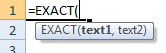(Notice how the formula inputs appear)

## EXACT Function Syntax and Inputs:

text1 – A string of text.

text2 – A string of text.

## How to use the EXACT Function in Excel:

The EXACT function checks if two text strings are well… exactly the same. This includes the case (upper or lower) of all letters. It returns a TRUE if they are the same, and FALSE if they are not.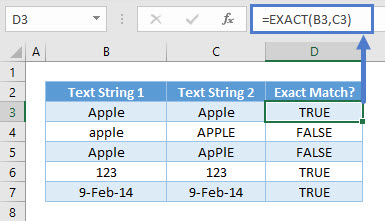As seen above, when comparing “apple”, TRUE is only returned when all cases match.

Numbers in row 6 and dates (which are stored as numbers) in row 7 won’t have issues with case sensitivity. They just have to be the same number or date to return TRUE.

## Check If Text in Upper Case

You might require users of a file to type an ID in upper-case. Use this formula to check that they’ve correctly entered text in upper-case: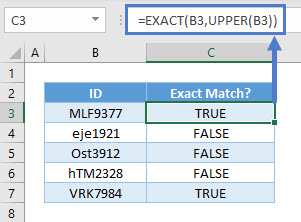UPPER converts all characters in ID to upper casing. Using that, you can compare if the original ID typed in B3:B7 to the ID with all upper casing. This formula can also be placed in a Data Validation to stop them from entering in the first place or in Conditional Formatting to highlight that it’s wrong.

## Case-Sensitive COUNTIF

COUNTIF counts the number of cells that meet a criteria. It is NOT case-sensitive.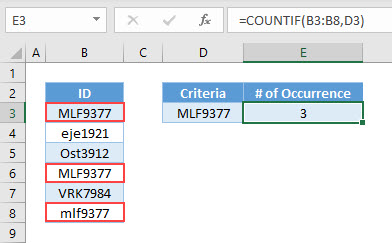Notice in the above example COUNTIF returns 3, which includes the lower case “mlf9377”.

To perform a case-sensitive COUNTIF, use the SUMPRODUCT and EXACT Functions instead: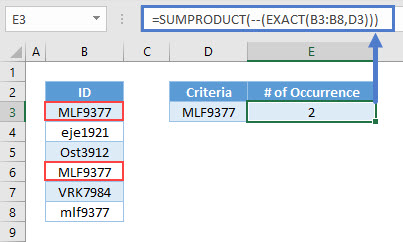## EXACT Function in Google Sheets

The EXACT Function works exactly the same in Google Sheets as in Excel: